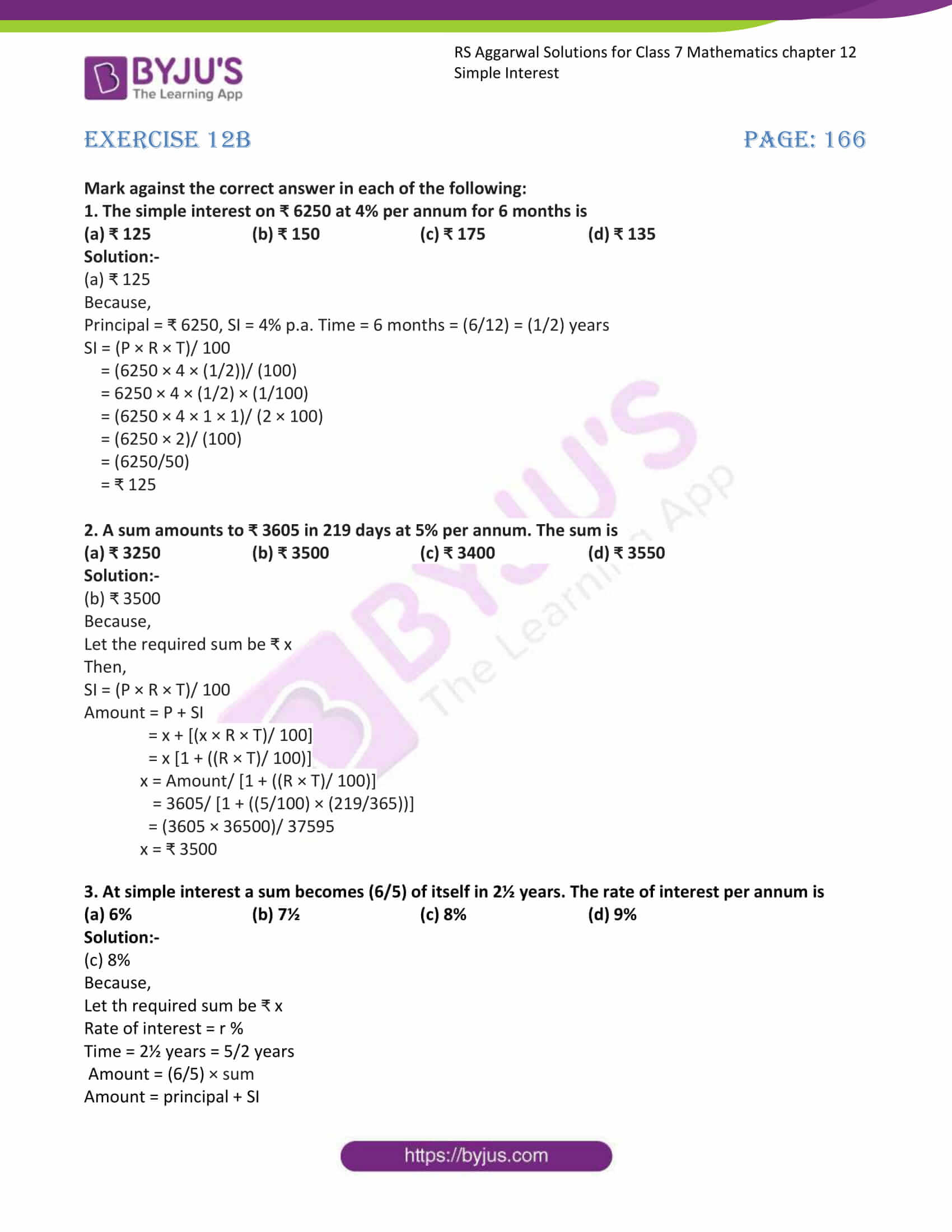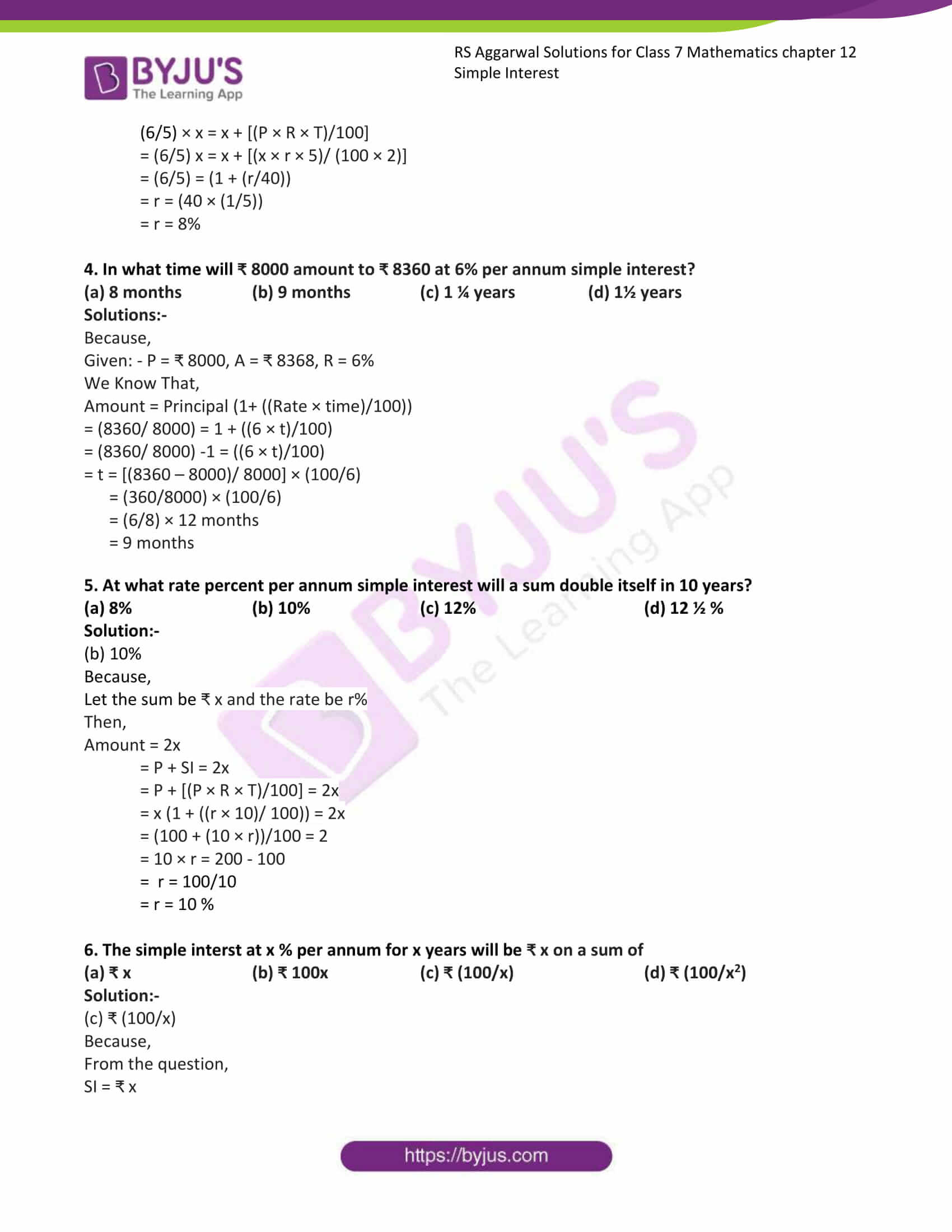# RS Aggarwal Solutions for Class 7 Maths Exercise 12B Chapter 12 Simple Interest

RS Aggarwal Solutions for Class 7 Maths Exercise 12B Chapter 12 Simple Interest in simple PDF are available here. This exercise of RS Aggarwal Solutions for Class 7 Chapter 12 contains objective type questions. It covers all the topics in the chapter with different kinds of questions. Students who go through these questions can be able to solve any type of questions. Students are suggested to try solving the questions from RS Aggarwal Solutions for Class 7 Maths Chapter 12 Simple Interest.

## Download the PDF of RS Aggarwal Solutions For Class 7 Maths Chapter 12 Simple Interest – Exercise 12B### Access answers to Maths RS Aggarwal Solutions for Class 7 Chapter 12 – Simple Interest Exercise 12B

Mark against the correct answer in each of the following:

1. The simple interest on ₹ 6250 at 4% per annum for 6 months is

(a) ₹ 125 (b) ₹ 150 (c) ₹ 175 (d) ₹ 135

Solution:-

(a) ₹ 125

Because,

Principal = ₹ 6250, SI = 4% p.a. Time = 6 months = (6/12) = (1/2) years

SI = (P × R × T)/ 100

= (6250 × 4 × (1/2))/ (100)

= 6250 × 4 × (1/2) × (1/100)

= (6250 × 4 × 1 × 1)/ (2 × 100)

= (6250 × 2)/ (100)

= (6250/50)

= ₹ 125

2. A sum amounts to ₹ 3605 in 219 days at 5% per annum. The sum is

(a) ₹ 3250 (b) ₹ 3500 (c) ₹ 3400 (d) ₹ 3550

Solution:-

(b) ₹ 3500

Because,

Let the required sum be ₹ x

Then,

SI = (P × R × T)/ 100

Amount = P + SI

= x + [(x × R × T)/ 100]

= x [1 + ((R × T)/ 100)]

x = Amount/ [1 + ((R × T)/ 100)]

= 3605/ [1 + ((5/100) × (219/365))]

= (3605 × 36500)/ 37595

x = ₹ 3500

3. At simple interest a sum becomes (6/5) of itself in 2½ years. The rate of interest per annum is

(a) 6% (b) 7½ (c) 8% (d) 9%

Solution:-

(c) 8%

Because,

Let th required sum be ₹ x

Rate of interest = r %

Time = 2½ years = 5/2 years

Amount = (6/5) × sum

Amount = principal + SI

(6/5) × x = x + [(P × R × T)/100]

= (6/5) x = x + [(x × r × 5)/ (100 × 2)]

= (6/5) = (1 + (r/40))

= r = (40 × (1/5))

= r = 8%

4. In what time will ₹ 8000 amount to ₹ 8360 at 6% per annum simple interest?

(a) 8 months (b) 9 months (c) 1 ¼ years (d) 1½ years

Solutions:-

Because,

Given: – P = ₹ 8000, A = ₹ 8368, R = 6%

We Know That,

Amount = Principal (1+ ((Rate × time)/100))

= (8360/ 8000) = 1 + ((6 × t)/100)

= (8360/ 8000) -1 = ((6 × t)/100)

= t = [(8360 – 8000)/ 8000] × (100/6)

= (360/8000) × (100/6)

= (6/8) × 12 months

= 9 months

5. At what rate percent per annum simple interest will a sum double itself in 10 years?

(a) 8% (b) 10% (c) 12% (d) 12 ½ %

Solution:-

(b) 10%

Because,

Let the sum be ₹ x and the rate be r%

Then,

Amount = 2x

= P + SI = 2x

= P + [(P × R × T)/100] = 2x

= x (1 + ((r × 10)/ 100)) = 2x

= (100 + (10 × r))/100 = 2

= 10 × r = 200 – 100

= r = 100/10

= r = 10 %

6. The simple interst at x % per annum for x years will be ₹ x on a sum of

(a) ₹ x (b) ₹ 100x (c) ₹ (100/x) (d) ₹ (100/x2)

Solution:-

(c) ₹ (100/x)

Because,

From the question,

SI = ₹ x

Rate = x % .p.a.

Time = x years

Then,

SI = (P × R × T)/ 100

X = (P × X× X)/ 100

P = (100X)/ (X × X)

P = ₹ (100/X)

### Access other exercises of RS Aggarwal Solutions For Class 7 Chapter 12 – Simple Interest

Exercise 12A Solutions